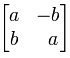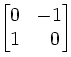# In defense of j

I’m about to commit an act of mathematical heresy.

I am a complex analyst by trade, which means I am a mathematician who studies the properties and utility of using complex numbers in calculus operations. Complex numbers, you might recall, are numbers of the form a + b i, where a and b real numbers and i is the imaginary number; i.e. i =$sqrt{-1}$. Complex numbers are essential tools of mathematics. Complex numbers provide natural and beautiful ways to connect arithmetic and geometry, algebra and trigonometry, differentiation and integration, single-variable and multi-variable calculus, number theory and analysis, mathematics with genetics… you name it.

The problem, however, is that no one outside of trained and dedicated mathematicians seems to know this. I myself have taught Complex Analysis as a class a number of times, and each time I found that after fifteen weeks of showing students how the use of complex numbers fundamentally unifies almost all of the concepts they’ve learned about in their other math class, how they provide novel and easy ways to solve problems raised in those other classes, and then how it goes on to produce results of surprising beauty and elegance and utility in its own right…. even after all that, I still have a nontrivial subset of students who will say “That’s nice and all, but it isn’t real. It’s still all imaginary numbers.”

That stupid phrase imaginary has done more to damage the perception of complex numbers than anything else I can think of. I mean, by its very definition, the word imaginary describes something that doesn’t exist or, at the very best, something utterly useless. You don’t see this self-destructive naming convention in the other sciences. Physicists don’t call gravity the phantom suck; chemists don’t mix solutions with the element doesnotexistium; biologists haven’t relabeled the appendix as the useless organ, even though it’s clearly more accurate.

Mathematicians tried to fix this somewhat by changing the name of imaginary numbers to complex numbers, although in hindsight the decision to change the adjective from one that describes something that does not exist to one that describes something that’s ungodly difficult wasn’t probably the optimal fix. No, I have a better solution.

It’s time to retire Euler’s choice of the letter i for the imaginary number$sqrt{-1}$.

I’m serious.

The problem with using i is that it stands for a number that is, well, imaginary. It’s a self-defeating prophecy. Students are quite capable of appreciating the use of$pi$ (pi) in  geometry without necessarily knowing that is the first letter in the Greek word for “perimeter.” Students are quite capable of appreciating the use of e in calculus and calling it the base of the natural logarithm without necessarily ever knowing that it’s named for a Swiss mathematician whose name sounds like “Oiler.” So why can’t we give students a chance  to appreciate the use of i in algebra… and geometry!… and calculus!… without first telling them what the letter stands for.

We need to get rid of it.

I’m not suggesting that we go back to the$sqrt{-1}$ notation of yore — that’s a choice frought with peril. For a positive real number x, the radical symbol$sqrt{x}$ refers specifically to the unique non-negative number r such that the product of r with itself is x, and given this definition, we have a number of important rules of radicals, such as$big( sqrt{x} big) cdot big( sqrt{y} big) = sqrt{x cdot y}$.

However, even if we accept the existence of complex numbers (and I do!), there is no way to properly define$sqrt{-1}$, since there is no unique non-negative root of -1, as both i and 1/i are such roots. And even if we could somehow unambiguously define what complex number we meant by$sqrt{-1}$, it still wouldn’t respect the previously established rules of radicals. As a simple example, if the rules of radicals applied to the root of -1, we could conclude that$-1 = big( sqrt{-1} big) big(sqrt{-1} big) = sqrt{(-1)(-1)} = sqrt{1} = 1.$

No, no, no… I have a better idea.

We should use the letter j instead of i.

Bear with me. I submit three criteria by which j is a better choice.

First: j is a neutral letter. It doesn’t stand for anything in general, and in particular it doesn’t stand for imaginary. Instead, we just say that j denotes the complex unit, a number that multiplies against itself to yield -1.

Second: the letter j arises naturally from vector calculus. To be specific, the standard modern definition of the complex numbers is essentially to identify the complex number$a + b ,sqrt{-1}$ with the 2-dimensional vector (a,b) in the Euclidean plane$mathbb{R}^2$. Vectors already have their own addition, whereas a “complex” vector product can be found by multiplying lengths and adding angles. With these definitions it can be shown that

(a,b)(c,d) = (ac – bd, ad + bc )

for any vectors (a,b) and (c,d) in the plane.   In particular,  this implies that

(a,b) = (a,0)(1,0) + (b,0)(0,1)

for any real numbers a and b. Since we commonly identify the x-axis of the plane with the real line, it is equally common to identify a complex number realized as the vector (x,0) with the real number x. In this case, the equation above can be written as

(a,b) = a (1,0) + b (0,1).

In vector calculus, we usually denote the vector (1,0) by i and the vector (0,1) by j. Thus, every complex number/plane vector can be written in the form

(a,b) = a i + b j,

which complete agrees with any student’s calculus intuition (although the calculus version is thinking of these as vector scalings rather than complex vector products).

Of course, the identification above also implies that the vector (1,0) is identified with the real number 1, so the equation above can equivalently be expressed as saying that any complex number can be written as

(a,b) = a + b j,

which looks exactly like the standard form of a complex number with the vector j standing in for$sqrt{-1}$. Even more compelling, note that

j2 = j j = (0,1) (0,1) = (-1, 0) = -1,

so j is indeed a square root of -1. Consequently, it makes sense to use j to denote the complex unit, particularly when we want to think of it as a number rather than a vector.

Third: I refers to multiplicative identities. Note that my argument above implied that the real number 1 is identified with the vector i; and if we further follow the font-style convention suggested above, we would also write 1 as the complex number i.

That may look a little weird, but it ain’t a bad idea. In fact, it’s entirely consistent with another naming convention students are likely to see (at least in part) in vector calculus. In the ring of square matrices, the unique multiplicative identity is denoted I; that is, it is the unique matrix element such that A I = I A = A for any matrix A. It turns out that a second common construction of the complex numbers is to identify the complex number a + b$sqrt{-1}$ with the 2 x 2 matrixwith the usual matrix addition and matrix multiplication. In particular, the complex unit is represented by the matrixwhich could be conceivably denoted by any letter (like J), while the real number 1 is identified with the matrixwhich is the identity matrix, and is universally denoted by I. Hence, identifying 1 with I is in fact required when we use the matrix description of complex numbers (which is crucial in articulating the connection between complex differentiability and vector-field differentiability).

Thus, I respectfully submit that henceforth we banish the term imaginary and with it, that horrible perpetrator of confusion and distrust, the letter “i.” In its place, let us choose only to speak of the complex number j, and…

Wait… what? Electrical engineers have been doing this for ages?

Oh. Well, then, false alarm. Stupid idea.

This entry was posted in mathify. Bookmark the permalink.